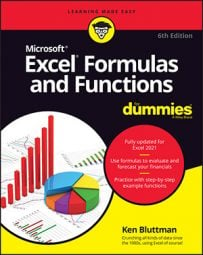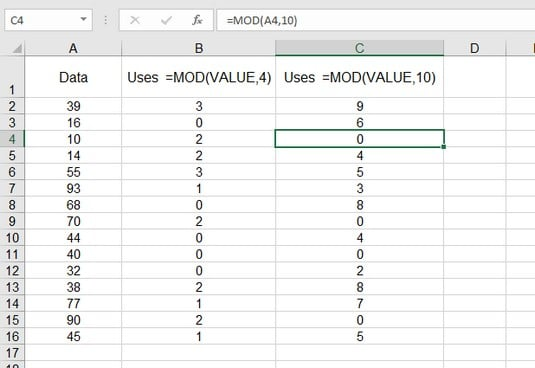##### Excel Formulas & Functions For DummiesThe Excel MOD function returns the remainder from an integer division operation. This remainder is called the modulus, hence the function’s name. The Excel MOD function has two arguments: the number being divided and the number being used to divide the first argument. The second argument is the divisor. The syntax for the Excel MOD function follows:
`=MOD(number, divisor)`
These are examples of the MOD function:

`=MOD(12,6)` returns 0.

`=MOD(14,5)` returns 4.

`=MOD(27,7)` returns 6.

`=MOD(25,10)` returns 5.

`=MOD(25,-10)` returns –5.

`=MOD(15.675,8.25)` returns 7.425.

The returned value is always the same sign as the divisor.

You can use MOD to tell whether a number is odd or even. If you simply use a number 2 as the second argument, the returned value will be 0 if the first argument is an even number and 1 if it is not.

But what's so great about that? You can just look at a number and tell whether it’s odd or even. The power of the MOD function is apparent when you’re testing a reference or formula, such as `=MOD(D12 - G15,2)`. In a complex worksheet with many formulas, you may not be able to tell when a cell will contain an odd or even number.

Taking this a step further, the Excel MOD function can be used to identify cells in a worksheet that are multiples of the divisor.Using MOD to find specific values.

Row 1 of the worksheet in the image above shows examples of the formulas that are entered in the successive rows of columns B and C, starting from the second row. Column A contains numbers that will be tested with the MOD function. If you’re looking for multiples of 4, the MOD function has 4 as the divisor, and when a value is a multiple of 4, MOD returns 0. This is evident when you compare the numbers in column A with the returned values in column B.

The same approach is used in column C, only here the divisor is 10, so multiples of 10 are being tested for in column A. Where a 0 appears in column C, the associated number in column A is a multiple of 10.

In this way, you can use Excel’s MOD function to find meaningful values in a worksheet.